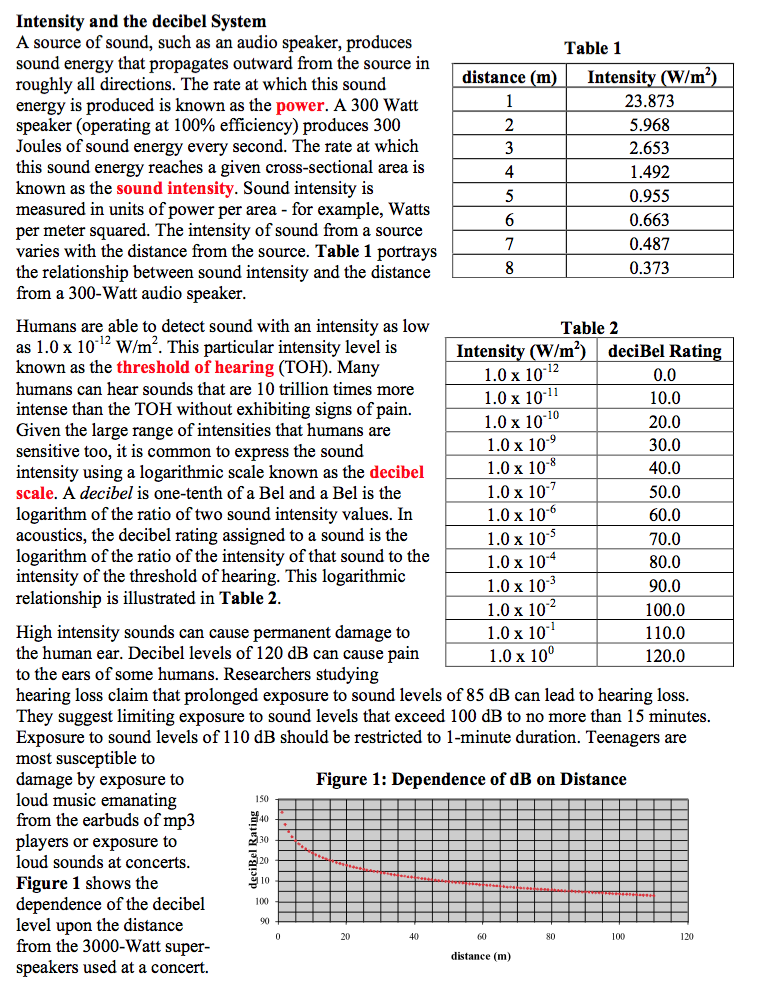###Intensity and the DeciBel System

Resource:
The Science Reasoning Center: Intensity and the Decibel System

Grade Level: High School

Description:
This passage describes the relationship between the intensity of a sound at a given distance from the source and the associated decibel level. Information is presented in the form of two tables and a graph. Questions target a student's ability to recognize the qualitative and quantitative relationship between variables, to use the relationship to compare various sounds with different intensities or decibel ratings or at different distances from the same source, and to extrapolate from a table or a graph to predict decibel ratings or intensities for a given set of conditions.

Performance Expectation:
HS-PS3-2  Develop and use models to illustrate that energy at the macroscopic scale can be accounted for as a combination of energy associated with the motions of particles (objects) and energy associated with the relative positions of particles (objects).

This activity aligns with the three dimensions of the Next Generation Science Standards in the manner described below:

Disciplinary Core Ideas
 Definitions of Energy (HS-PS3.A.2):  At the macroscopic scale, energy manifests itself in multiple ways, such as in motion, sound, light, and thermal energy. A source of sound, such as an audio speaker, produces sound energy that propagates outward from the source in roughly all directions.  The rate at which this sound energy reaches a given cross-sectional area is known as the sound intensity. This passage explores how sound intensity is measured.  And since the intensity of sound from a source varies with the distance from the source, this passage also familiarizes the reader with the inverse squared relationship that exists between sound intensity and distance.

Crosscutting Concepts
 Scale, Proportion, and Quantity:  The significance of a phenomenon is dependent on the scale, proportion, and quantity at which it occurs. Humans are able to detect sound intensities that span an incredible range—approximately 12 orders of magnitude!  Given this large range of intensities, it is common to express sound intensity using a logarithmic scale known as the decibel system.  A data presentation in this passage illustrates how the decibel rating assigned to a sound is the logarithm of the ratio of the intensity of that sound to the intensity of the threshold of hearing (TOH).  With such a range of intensities spanning many orders of magnitude, this passage provides a great example of how this cross-cutting concept may be addressed in physics. Energy and Matter:  Energy cannot be created or destroyed—it only moves between one place and another place, between objects and/or fields, or between systems. By using the example of a high wattage music speaker, the reader can understand how electrical energy transfers to mechanical energy (the vibration of the speaker head) and then to sound energy as the vibration of air molecules.  In turn, as a fraction of this sound energy reaches a given cross-sectional area, it is able to be detected by the human ear.  Implicit in this example is the underlying principle that as energy is transferred from one form to another (and from one place to another), no energy was created or destroyed in this process.

Practices
 Analyzing and Interpreting Data: Analyze data using computational models in order to make valid and reliable scientific claims. Two data table and one graph provide a data-rich presentation for students to explore.  While some questions at the end of the passage require students to analyze a single data presentation, many of the questions require students to combine the results of one data presentation with another.  This higher level analysis skill is an important one for all scientists to make valid and reliable claims about complex systems. Using Mathematical and Computational Thinking:  Use mathematical representations of phenomena to describe explanations. In this passage students see how describing something as common as sound and hearing requires one to be familiar with both logarithmic and inverse squared relationships.  As they do, students are then asked to apply these mathematical relationships in order to describe sound intensities at various distances from a sound source, to compare the decibel level of one sound intensity to that of another, and to combine these ideas in order to understand how the decibel level varies with distance.  Few other physics phenomena provide such easy access to these mathematical and computational relationships.

Associated Reading from The Physics Classroom

Other Supporting Pages at The Physics Classroom:

View Infographic.
(Coming Soon)

### Search the NGSS Corner

Maybe you're looking for something really specific that pertains to a desired topic and emphasizes one or more of the listed NGSS dimensions. Why not try a search of this section of our website? Simply select from one or more of the pull-down menus and click Search  This page will reload and a collection  of possibilities will be displayed in this section of the page and sorted by relevancy.

Follow Us